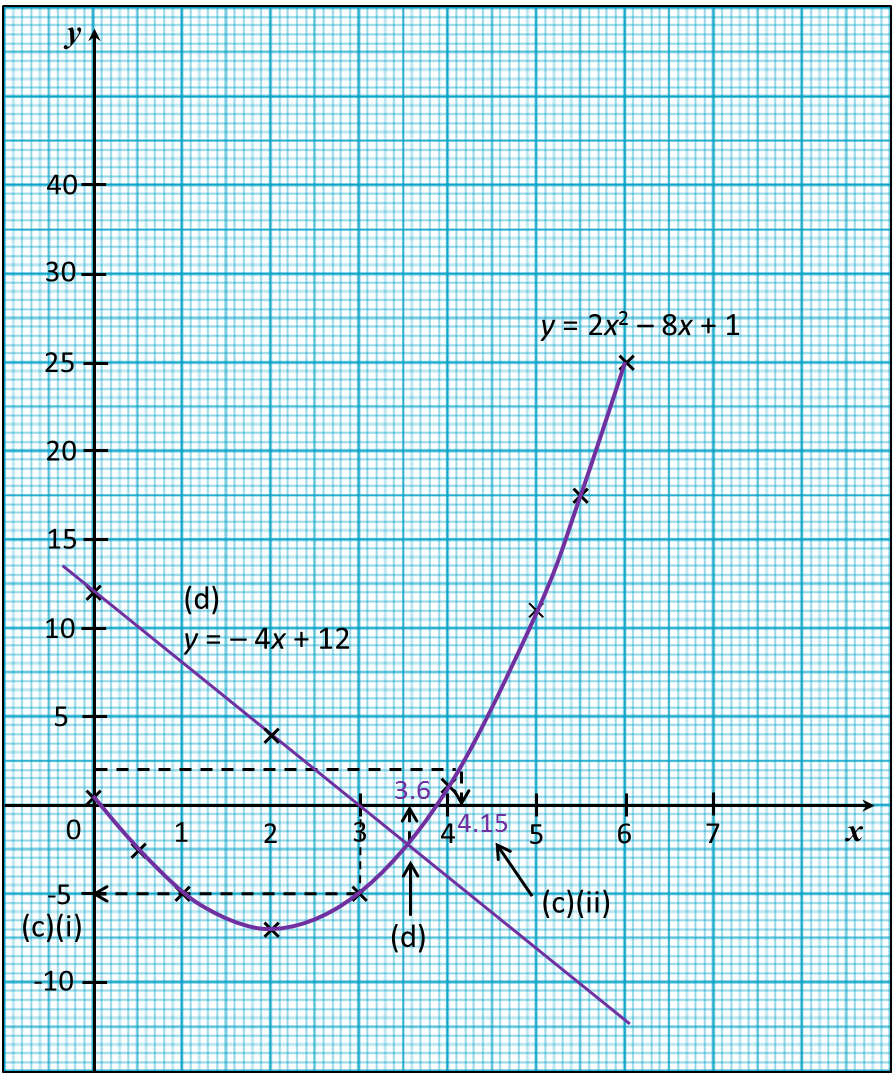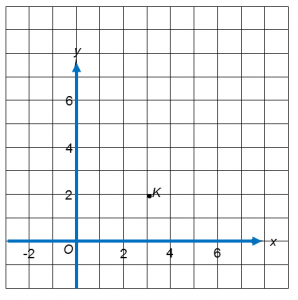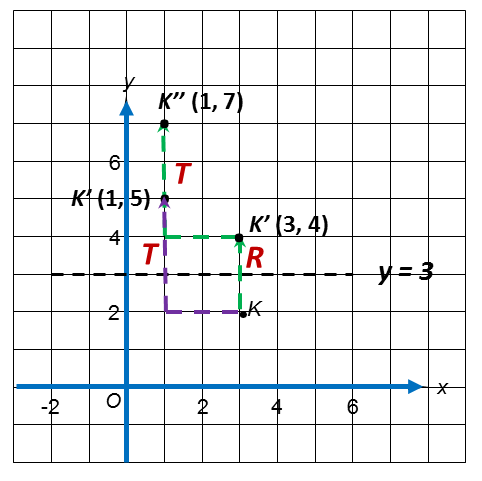# SPM Mathematics (Model Test Paper), Question 12 & 13

SPM Mathematics (Model Test Paper)

Section B
[48 marks]
Answer any four questions from this section.

12 (a)
Complete Table 1 in the answer space for the equation y = 2x2− 8x + 1.
[2 marks]
(b) For this part of the question, use the graph paper provided. You may use a flexible curve rule.
By using a scale of 2 cm to 1 unit of the x-axis and 2 cm to 5 units on the y-axis, draw the graph of y = 2x2− 8x + 1 bagi 0 ≤ x ≤ 6.  [4 marks]
(i)   the value of y when x = 3,
(ii)   the value of x when y = 2,  [2 marks]
(d) Draw a suitable straight line on your graph to find all the values of x which satisfy the equation 2x2− 4x − 11 = 0 for 0 ≤ x ≤ 6. State the values of x.   [4 marks]
(a)
 x 0 0.5 1 2 3 4 5 5.5 6 y 1 −2.5 −5 −7 −5 1 25
Table 1
(a)
 x 5 5.5 y 11 17.5
(b)(c)(i)
From the graph, when x = 3, = −5

(c)(ii)
From the graph, when y = 2, = 4.15

(d)
y = 2x2 − 8x + 1 ---- (1)
2x2 − 4x− 11 = 0
0 = 2x2 − 4x– 11 ---- (2)
(1) minus (2),
The suitable straight line is y = −4x + 12

 x 0 2 y 12 4

From the graph, x = 3.6

13. (a)  Diagram 8.1 shows point K ( 3, 2 ) marked on a Cartesian plane.Diagram 8.1
The transformation T is a translation $\left(\begin{array}{l}-2\\ \text{}3\end{array}\right).$
The transformation R is a reflection in the line y = 3.

State the coordinates of the image of point K under each of the following transformations.
(i)   T
(ii)  TR [3 marks]

(b)  Diagram 8.2  shows three trapeziums, ABCD, PQRS and PTUV drawn on a Cartesian plane.Diagram 8.2

(i) Trapezium PTUV is the image of trapezium ABCD under the combined transformation VW
Describe in full, the transformation W and the transformation V.  [ 6 marks]
(ii) Given that the area of trapezium ABCD is 14 cm2, calculate the area, in cm2, of the shaded region. [3 marks](a)(i) (3, 2) → T → (1, 5)

(a)(ii)
(3, 2) → R → (3, 4) → T → (1, 7)

(b)(i)W : Rotation, 90o clockwise at centre (0 , −1)
V: Enlargement, scale factor of 2, centre of enlargement at point P (2 , 1)
(ii)
Area of PTUV = (scale factor)2 × Area of object
=  22  × 14
= 56 cm2
Area of shaded region = 56 – 14 = 42 cm2